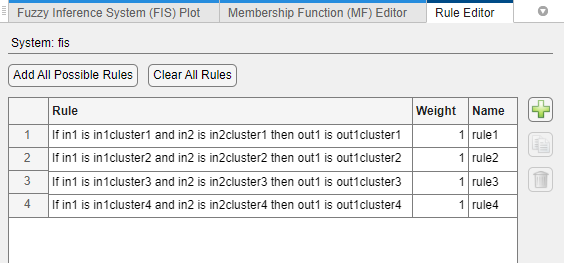## Get Started Using Fuzzy Logic Designer

After you open the Fuzzy Logic Designer app, you can:

• Open an existing fuzzy inference system (FIS) from the MATLAB® workspace or a FIS file (`*.fis`).

• Create a template FIS structure based on the number of input and output variables for your application.

• Create a FIS based on input and output data.After you open a FIS or create a new FIS structure, you must configure your system by defining:

After configuring your FIS, you can analyze its behavior within the app. For more information, see Analyze Fuzzy System Using Fuzzy Logic Designer.

For an example that shows how to create, configure, and analyze a fuzzy inference system, see Build Fuzzy Systems Using Fuzzy Logic Designer.

### Open Existing FIS

You can open an existing FIS from MATLAB workspace or a FIS file.

To open a FIS from the MATLAB workspace, in the Open from Workspace drop-down list, select the FIS.To open a FIS from a FIS file (`*.fis`), click Browse. Then, in the Open Fuzzy Inference System dialog box, browse to the folder that contains the file, select the file, and click .

### Create Template FIS Structure

You can create a template FIS structure for any of the supported FIS types.

• Type-1 Mamdani system

• Type-2 Mamdani system

• Type-1 Sugeno system

• Type-2 Sugeno system

If your application has two input variables and one output variable, in the Getting Started dialog box, under Template Fuzzy Inference Systems, click the corresponding type of FIS that you want to create.If your application has more than two inputs or more than one output, in the Getting Started dialog box, under General Fuzzy Inference Systems, click Custom FIS.

In the Custom System dialog box, select the type of FIS that you want to create and specify the FIS name and the number of input and output variables.When you create a template FIS structure, each input variable has three triangular membership functions. For Mamdani systems, each output variable also has three triangular membership functions. For Sugeno systems, each output variable has three constant membership functions.

Each input and output variable has a default range of 0 through 1.

### Create FIS from Data

If you have input/output data that spans the operating ranges for your system variables, you can create a FIS based on clusters derived from this data. To create a FIS from data, in the Getting Started dialog box, under General Fuzzy Inference Systems, click FIS from Data.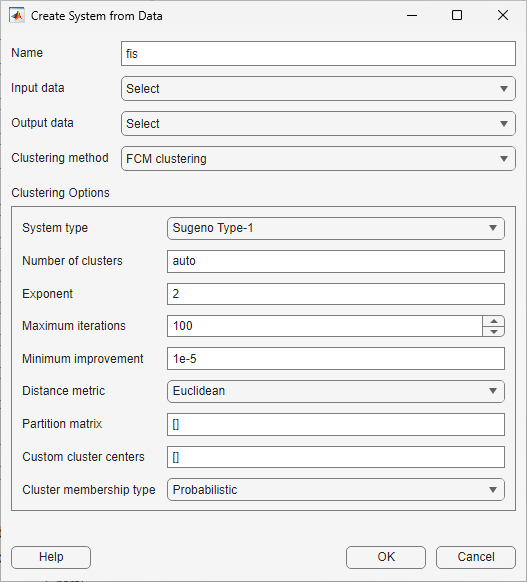In the Create System from Data dialog box, select the input and output data using the Input data and Output data drop-down lists, respectively. Each list displays any valid numerical arrays available in the MATLAB workspace.

When you select data consider the following:

• Input data must be an N-column numerical array, where N is the number of FIS inputs.

• Output data must be an M-column array, where M is the number of FIS outputs.

• When using the grid partition clustering method, the output data must have one column. If you specify data with more than one column for grid partitioning, the app uses only the first column as the output data.

• The input and output data arrays must have the same number of rows.

Then, select the type of clustering using the Clustering method drop-down list.

#### FCM Clustering

You can generate a Mamdani or Sugeno fuzzy system using membership functions derived from data clusters found using FCM clustering of input and output data.

• Each input and output variable contains one membership function for each cluster.

• Input variables use Gaussian membership functions.

• For Mamdani systems, the output variables use Gaussian membership functions.

• For Sugeno systems, the output variables use linear membership functions.

To configure the FCM clustering, use the options shown in the following table.

ParameterDescription
System type

Fuzzy inference system type, specified as one of the following values.

• `Sugeno Type-1`

• `Mamdani Type-1`

Number of clusters

Number of clusters to create, specified as `auto` or an integer greater than 1. When you specify `auto`, the app estimates the number of clusters using subtractive clustering.

Exponent

Exponent for the fuzzy partition matrix, specified as a scalar greater than 1. This option controls the amount of fuzzy overlap between clusters, with larger values indicating a greater degree of overlap.

Maximum iterations

Maximum number of FCM iterations, specified as a positive integer.

Minimum improvementMinimum improvement in the objective function between two consecutive iterations, specified as a positive scalar.
Distance Metric

Method for computing distance between data points and cluster centers, specified as one of the following values.

• `Euclidean` — Compute distance using a Euclidean distance metric, which corresponds to the classical FCM algorithm.

• `Mahalanobis` — Compute distance using a Mahalanobis distance metric, which corresponds to the Gustafson-Kessel FCM algorithm.

#### Grid Partition

You can generate input membership functions by uniformly partitioning the input variable ranges, and create a single-output Sugeno fuzzy system with one membership function for each possible input variable combination. You can specify the membership function type for the input and output variables.

To configure the FCM clustering, use the options shown in the following table.

ParameterDescription
Input membership function typeInput membership function type. For more information on the types of membership functions, see Foundations of Fuzzy Logic.
NumberNumber of membership functions to use for input variables, specified as an integer greater than 1.
Output membership function typeOutput membership function type, specified as either `Linear` or `Constant`.

By default, the app uses the same membership function settings for all input variables. However, you can use a different membership function number and type for each input variable. To do so, clear the Use same membership function settings for each input parameter.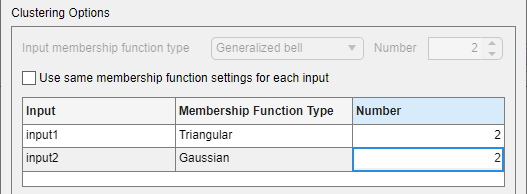#### Subtractive Clustering

You can generate a Sugeno fuzzy system using membership functions derived from data clusters found using subtractive clustering of input and output data.

• Each input and output variable contains one membership function for each cluster.

• Input variables use Gaussian membership functions.

• Output variables use linear membership functions.

Each input and output variable contains one membership function for each cluster. For more information on the subtractive clustering algorithm, see `subclust`.

To configure the clustering, use the options shown in the following table.

ParameterDescription
Cluster influence range

Range of influence of the cluster center for each input and output assuming the data falls within a unit hyperbox, specified as one of the following values.

• Scalar value in the range [0 1] — Use the same influence range for all inputs and outputs.

• Vector — Use different influence ranges for each input and output.

Specifying a smaller range of influence usually creates more and smaller data clusters.

Data scale

Data scale factors for normalizing input and output data into a unit hyperbox, specified as a 2-by-N array, where N is the total number of inputs and outputs. Each column specifies the minimum value in the first row and the maximum value in the second row for the corresponding input or output data set.

When the data scale is `auto`, the app uses the actual minimum and maximum values in the data to be clustered.

Squash factor

Squash factor for scaling the range of influence of cluster centers, specified as a positive scalar. A smaller squash factor reduces the potential for outlying points to be considered as part of a cluster, which usually creates more and smaller data clusters.

Accept ratio

Acceptance ratio, defined as a fraction of the potential of the first cluster center, above which another data point is accepted as a cluster center, specified as a scalar value in the range [0 1]. The acceptance ratio must be greater than the rejection ratio.

Reject ratio

Rejection ratio, defined as a fraction of the potential of the first cluster center, below which another data point is rejected as a cluster center, specified as a scalar value in the range [0 1]. The rejection ratio must be less than acceptance ratio.

Custom cluster centers

Custom cluster centers, specified as a C-by-N array, where C is the number of clusters and N is the total number of inputs and outputs.

To automatically compute cluster centers, set the custom centers to `[]`.

### Automatically Generate Rules

When you create a FIS, you can automatically populate the rule base. To do so, before creating the FIS, select Generate rules automatically. By default, this option is selected.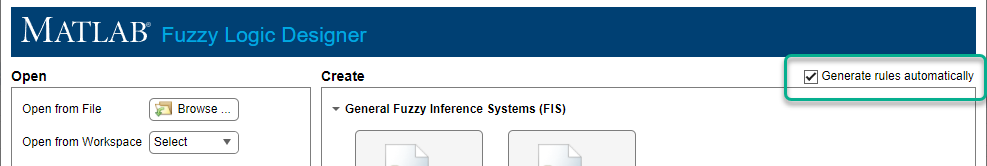What rules are generated depend on how you create the FIS.

#### Rules for FIS Template Structure

When you generate rules for a FIS template structure, the app adds an AND-based rule for each possible combination of input membership functions. For example, the following figure shows the nine generated rules for a two-input system where each input variable has three membership functions.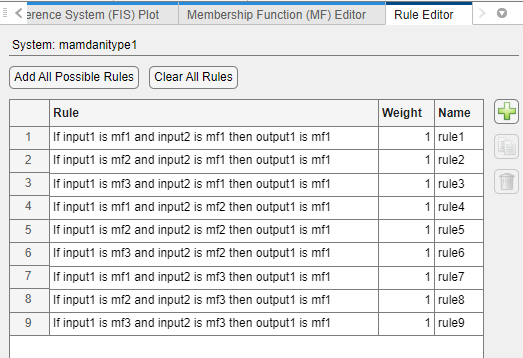For all of the generated rules, the default consequent is the first membership function of the first output variable.

#### FIS from Data

When you create a FIS from data, the generated rules depend on the type of clustering you select for creating your FIS.

• Grid partitioning — One AND-based rule for each input membership function combination. The consequent of each rule corresponds to a different output membership function. For example, the following figure shows the four generated rules for a two-input system where each input variable has two membership functions.• FCM or subtractive clustering — One AND-based rule for each fuzzy cluster. Each rule uses the cluster-specific membership function from each input and output variable. For example, the following figure shows the rules for a FIS with four clusters generated using FCM clustering.